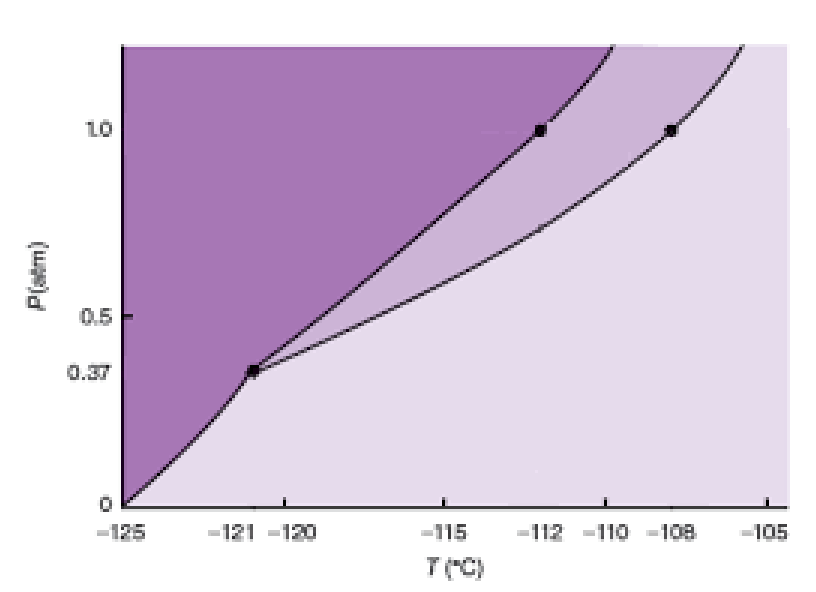# Problem: The phase diagram for xenon is shown below:If you measure the equilibrium vapor pressure of a liquid xenon sample to be 380 mm Hg, what is the temperature of the liquid phase?

###### FREE Expert Solution

Analyze the given phase diagram and determine temperature when the equilibrium vapor pressure is at 380 mmHg

Recall that a phase diagram shows the transition of matter between solid, liquid, and gas phases as temperature and pressure changes.

In a phase diagram, the different phases can be identified by their location:

• Solid: can be found at high pressure and low temperature

• Liquid: can be found between the solid and gas regions

• Gas: can be found at low pressure and high temperature

96% (36 ratings)###### Problem Details

The phase diagram for xenon is shown below:If you measure the equilibrium vapor pressure of a liquid xenon sample to be 380 mm Hg, what is the temperature of the liquid phase?

Frequently Asked Questions

What scientific concept do you need to know in order to solve this problem?

Our tutors have indicated that to solve this problem you will need to apply the Phase Diagram concept. You can view video lessons to learn Phase Diagram. Or if you need more Phase Diagram practice, you can also practice Phase Diagram practice problems.

What is the difficulty of this problem?

Our tutors rated the difficulty ofThe phase diagram for xenon is shown below:If you measure th...as medium difficulty.